# Selina Concise Solutions for Chapter 3 Fractions (including Problems) Class 7 ICSE Mathematics

##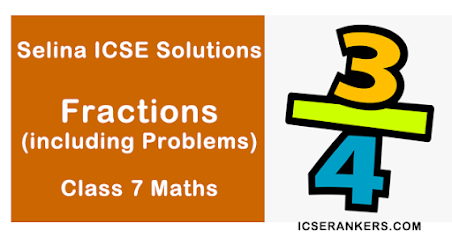### Exercise 3 (A)

1. Classify, each fraction given below, as decimal or vulgar fraction, proper or improper fraction and mixed fraction :

(i) 3/5
(ii) 11/10
(iii) 13/20
(iv) 13/7(vi) 19/103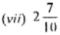(viii) 23/500

(i) Vulgar and Proper
(ii) Decimal and Improper
(iii) Decimal and Proper
(iv) Vulgar and Improper
(v) Mixed
(vi) Decimal
(vii) Mixed and Decimal
(viii) Vulgar and Proper Ans.

2. Express the following improper fractions as mixed fractions :
(i) 18/5
(ii) 7/4
(iii) 25/6
(iv) 38/5
(v) 22/5

3. Express the following mixed fractions as Improper fractions :

4. Reduce the given fractions to lowest terms

(i) 8/18
(ii) 27/36
(iii) 18/42
(iv) 35/75
(v) 18/45

5. State: true of false
(i) 30/40 and 12/16 are equivalent fractions.
(ii) 10/25 and 25/10 are equivalent fractions.
(iii) 35/49, 20/28, 45/63 and 100/140 arc equivalent

6. Distinguish each of the following fractions, given below, as a simple fraction or a complex fraction :

(i) 0/8
(ii) -3/-8
(iii) 5/-7

### Exercise 3 (B)

1. For each pair, given below, state whether it forms like fractions or unlike fractions
(i) 5/8 and 7/8
(ii) 8/15 and 8/21
(iii) 4/9 and 9/4
(i) 5/8 and 7/8 : These are like fractions.
(ii) 8/15 and 8/21 : These are unlike fractions.
(iii) 4/9 and 9/4 : These are unlike fractions.

2. Convert given fraction into fractions into fractions with equal denominators :
(i) 5/6 and 7/9
(ii) 2/3, 5/6 and 7/12
(iii) 4/5, 17/20, 23/40 and 11/16

3. Convert given fractions into fractions with equal numerators :
(i) 8/9 and 12/17
(ii) 6/13, 15/23 and 12/17
(iii) 15/19 , 25/28 , 9/11 and 45/47

4. Put the given fractions in ascending order by making denominators equal :
(i) 1/3, 2/5, 3/4 and 1/6
(ii) 5/6, 7/8, 11/12 and 3/10
(iii) 5/7, 3/8, 9/14 and 20/21

5. Arrange the given fractions in descending order by making numerators equal :
(i) 5/6, 4/15, 8/9 and i/3
(ii) 3/7, 4/9, 5/7 and 8/11
(iii) 1/10, 6/11, 8/11 and 3/5

6. Find the greater fraction :
(i) 3/5 and 11/15
(ii) 4/5 and 3/10
(iii) 6/7 and 5/9
(iv)3/8 and 4/9
(v) -2/7 and -3/10

7. Insert one fraction between :                                                                                                                  (i) 3/7 and 4/9
(ii) 2 and 8/3
(iii) 9/17 and 6/13

### Exercise 3 (C)

1. Reduce to a single fraction :

2. Simplify :
(vii) 2 ÷ 1/3
(viii) 3
÷ 2/5
(ix) 1
÷ 3/5
(x) 1/3
÷ 1/4
(xi) -5/8
÷ 3/4
3. Subtract :
(i) 2 from 2/3
(ii) 1/8 from 5/8
(iii) -2/5 from 2/5
(iv) -3/7 from 3/7
(v) 0 from -4/5
(vi) 2/9 from 4/5
(viii) -4/7 from -6/11

4. Find the value of
(i) 1/2 of 10 kg
(ii) 3/5 of 1 hour
(vi) 5/11 of 4/5 of 22 kg.

5. Simplify and reduce to a simple fraction :
(x) 4/5 ÷ 7/15 of 8/9
(xi) 4/5
÷ 7/15 × 8/9
(xii) 4/5 of 7/15
÷ 8/9
(xiii)1/2 of 3/4 × 1/2 + 2/3

7. Which is greater, 3/5 or 7/10 and by how much ?
Taking the cross multiplication, we get
3 × 10 = 30 and 7×5 = 35
Since, 3 × 10 (i.e., 30) is smaller than 7 × 5 (i.e.,)

For finding the required fraction, we have

∴ Area of the park = l × b
= 117/5 × 50/3
= 39 × 10 = 390 m2

15. Out of  24 kg of wheat, 5/6 th of wheat is consumed. Find, how much wheat is still left ?
Total wheat available = 24 kg
Wheat consumed = 5/6 th of 24 kg
= 5/6 × 24 = 20 kg
∴ Remaining wheat = 24 - 20 kg = 4 kg

16. A rod of lengthmetre is divided into five equal parts. Find the length of each part so obtained.
Length of rod to be divided into 5 equal parts.

(i) A + B
(ii) BA

3. 1/4(1/4 + 1/3) - 2/5

7. (1/2 + 2/3) ÷ (3/4 - 2/9)

### Exercise 3 (E)

1. A line AB is of length 6 cm. Another line CD is of length 15 cm. What fraction is :
(i) The length of AB to that of CD ?
(ii) 1/2 the length of AB to that of 1/3 of CD ?
(iii) 1/5 of CD to that of AB ?
Length of line AB = 6 cm
and length of line CD = 15 cm
(i) Length of AB to length of

2. Subtract 2/7 - 5/21 from the sum of 3/4, 5/7 and 7/12

No. of hours, a boy worked in 6 days

5. A student boughtof yellow ribbon,of red ribbon and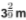of blue ribbon for decorating a room. How many metres of ribbon did he buy ?

6. In a business, Ram and Deepak invest 3/5 and 2/5 of the total investment. If Rs. 40,000 is the total investment, calculate the amount invested by each ?
Total investment = Rs. 40, 000
Ram's investment = 3/5 or Rs. 40, 000
= Rs. 3/5 × 40,000
= Rs. 3 × 8000 = Rs. 24, 000
Deepak's investment = 2/5 of Rs. 40,000
= Rs. 2/5 × 40,000
= Rs. 2 × 8000 = Rs. 16, 000

7. Geeta had  30 problems for home work. she worked out 2/5 of them. How many problems were still left to be worked out by her ?
No. of problems of Geeta = 30
No. of problems worked out = 2/3 of 30
= 2/3 ×30 = 20
No. of problems left out = 30 - 20 = 10

8. A picture was marked at Rs. 90. It was sold at 3/4 of its marked price. What was the sale price ?
Marked price = Rs. 90
Sale price = 3/4 of Rs. 90
= 3/4 × 90
= Rs. 270/4 = 67.50

9. Mani had sent fifteen parcels of oranges. What was the total weight of the parcels, if each weighed 21/2 kg ?
Total no. of parcels = 15
Weight of each parcel = 21/2 kg
Total weight = 15 of 21/2 kg = 21/2 × 15 kg
= 315/2 = 157.5 kg

11. The heights of two vertical poles, above the earth's surface, are 57/4 m and 67/3 respectively. How much higher is the second pole as compared with the height of the first pole ?
Height of one pole above earth's surface =57/4 and  height of second pole = 67/3
∴ Second pole is higher than the first pole
= 67/3 - 57/4
= (268 - 171)/ 12 = 97/12 m

12. Vijay weighed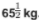. He gainedduring the first week,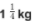during the second week, but lost 5/16 kg during the 16 third week. What was his weight after the third week ?

13. A man spends 2/5 of his salary on food and 3/10 on house rent, electricity, etc. What fraction of his salary is still left with him ?
Let salary of man = Re. 1
Amount spent on food = 2/5 or Re. 1 = Re. 2/5
and amount spent house rent  = 3/10 or Re. 1
= Re. 3/10

14. A man spends 2/5 of his salary on food and 3/10 of the remaining on house rent, electricity, etc. What fraction of his salary is still left with him ?
Let total amount of salary  = Re. 1
Amount spent on food = 2/5 of Re. 1
Remaining amount  = 1 - 2/5
= (5-2)/5 = Rs. 3/5
Amount spent on house rent etc.
= 3/10 of 3/5 = Rs. 9/50
Remaining amount left
= 3/5 - 9/50
= Rs (30- 9)/50 = Rs. 21/50
∴ Fraction of amount left = 21/50

15. Shyam bought a refrigerator for Rs. 5000. He paid 1/10 of the price in cash and the rest in 12 equal  monthly instalments. How much had he o pay each month ?
Total amount of the refrigerator  = Rs. 5000
Amount paid in cash  = 1/10 or Rs. 5000
= 1/10 × 5000 = Rs. 500
Balance amount = Rs. 5000 - Rs. 500
= Rs. 4500
No. of equally instalments  = 12
∴ Amount of each instalment
= Rs. 4500 ÷ 12 = Rs. 4500 × 1/12
= Rs. 375 Ans.

16. A lamp post has half of its length in mud, and 1/3 of its length in water.
(i) What fraction of its length is above the water ?
(ii) If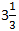m of the lamp post is above the water, find the whole length of the lamp post.
(i) Let length of the post = 1 m
then length of post in mud = 1/2 m
and length of post in water = 1/3 m
∴ Length of post above the water

17. I spent 3/5 of my savings and still have Rs. 2,000 left. What were my savings ?
Let my saving = 1, Part spent  = 3/5 of savings
∴ part left  = 1 - 3/5 = (5 -3)/5 = 2/5 of savings
But he left = Rs. 2000
∴ 2/5 of savings = Rs. 2000
∴ Total savings  = Rs. 2000 × 5/2
= Rs.  5000 Ans.

18. In a school, 4/5 of the children are boys. If the number of girls is 200, find the number of boys.
No. of boys = 4/5 of the total children
∴ No. of girls = (1 - 4/5) of total children
= (5-4)/5  = 1/5 of total children.
But no. of girls = 200
∴ 1/5 of total children = 200
Hence total number of children = 200 × 5/1 = 1000
∴ No. of boys = 4/5 of 1000 = 4/5 × 1000 = 800 Ans.

19. If  4/5 of an estate is worth Rs. 42, 000, find the worth of whole estate. Also, find the value of 3/7 of it.
4/5 of an estate = Rs. 42000
∴ Total value of estate = Rs. 42000 × 5/4
= Rs. 10500 × 5= 52500 Ans.
and value of 3/7 of it = 3/7 of its value
= 3/7 of  Rs. 52500 = 3/7 × 52500
= 3 × 7500 = Rs. 22500

20.  After going 3/4 of my journey, I find that I have covered 16 km. How much journey is still left ?
3/4 of journey = 16 km.

21.  When Krishna travelled 25 km, he found that 3/5 of his journey was still left. What was the length of the whole journey.
3/5 of the total journey was left
∴ Journey travelled by him  = 1 - 3/5
= (5-3)/ 5 =  2/5
∴ 2/5 of total journey  = 25 km
∴ Total journey  = 25 km × 5/2 = 125/2 km

22. From a piece of land, one - third is bought by Rajesh and one - third of remaining is bought by Manoj. If 600 m2  land is still left unsold, find the total area of the piece of land.
23 . A boy spent 3/5 of his money on buying 1 cloth and 1/4 of the remaining of buying shoes.  If initially he has 2400 ;  How much did he spend on shoes ?
Money in hand =  ₹ 2400
= 3/5 or ₹ 2400
= 3/5 × 2400 = 3 × 480 = ₹ 1440
Remaining money = ₹ 2400 - ₹ 1440 = ₹ 960
Now, boy spent 1/4 of the remaining money on buying shoes.
∴ Money spent on buying shoes = ₹ 960 × 1/4 = ₹240

24. A boy spent 3/5 of his money on buying cloth and 1/4 of his money on buying shoes.